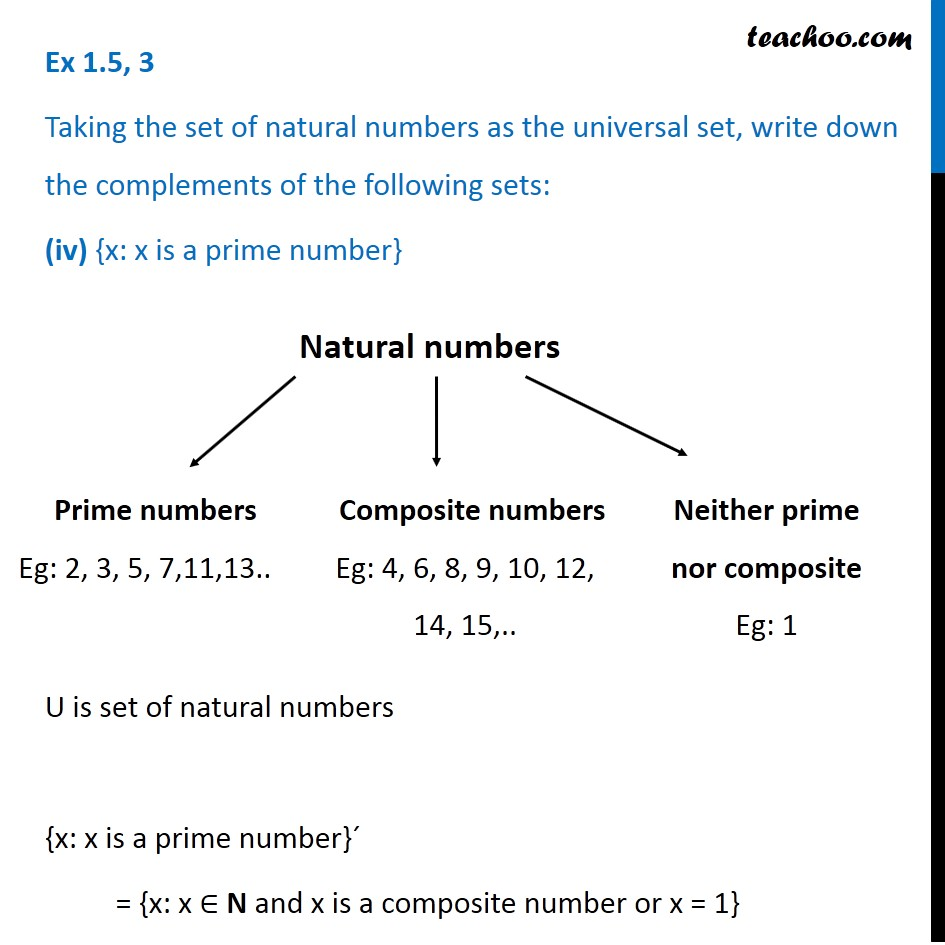1. Chapter 1 Class 11 Sets (Term 1)
2. Serial order wise
3. Ex 1.5

Transcript

Ex 1.5, 3 Taking the set of natural numbers as the universal set, write down the complements of the following sets: (iv) {x: x is a prime number} U is set of natural numbers {x: x is a prime number}´ = {x: x ∈ N and x is a composite number or x = 1} Composite numbers Eg: 4, 6, 8, 9, 10, 12, 14, 15,.. Neither prime nor composite Eg: 1

Ex 1.5

Chapter 1 Class 11 Sets (Term 1)
Serial order wise